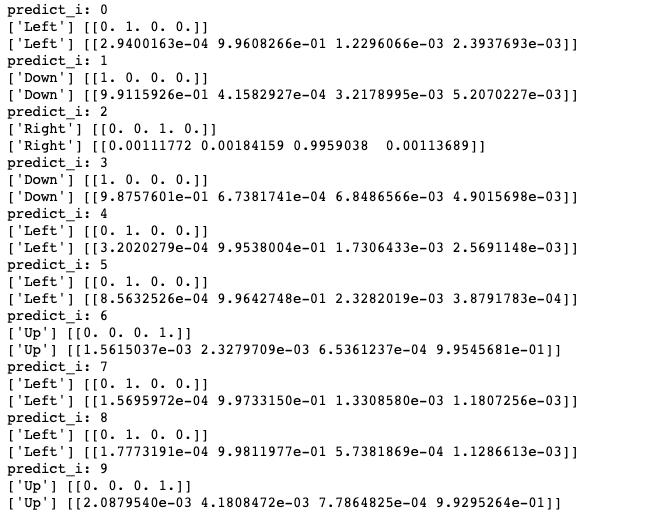The moving square video prediction problem is contrived to demonstrate the CNN LSTM. The problem involves the generation of a sequence of frames. In each image a line is drawn from left to right or right to left. Each frame shows the extension of the line by one pixel. The task is for the model to classify whether the line moved left or right in the sequence of frames.Technically, the problem is a sequence classification problem framed with a many-to-one prediction model.

"Moving Square Video Prediction"是《Long Short-Term Memory Networks With Python》 这本书里的一个示例。我在这里做了一下扩展，将其变成一个多分类问题。

### The Problem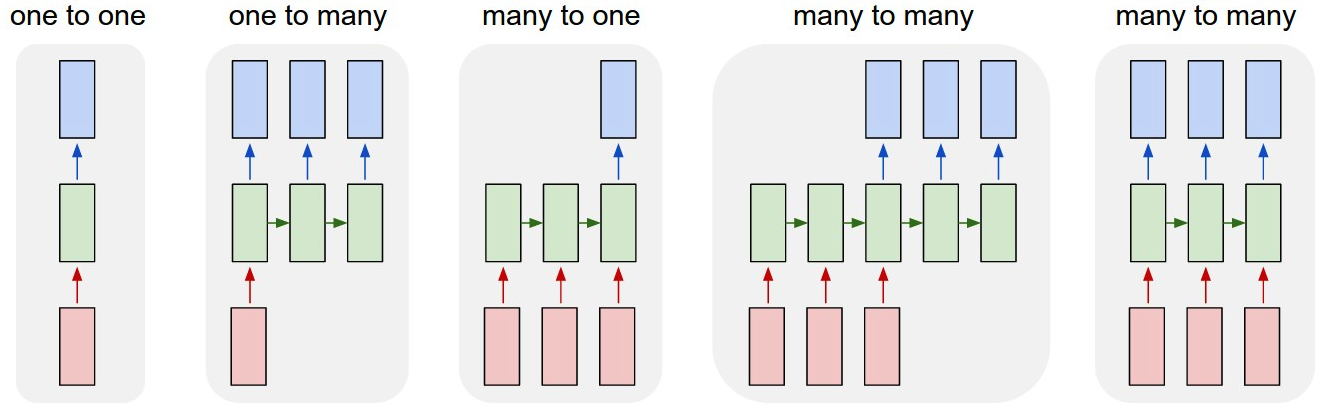### CNN LSTM

• 在其输入中具有空间结构，例如 2D 结构或图像中的像素或句子，段落或文档中的单词的一维结构。
• 在其输入中具有时间结构，诸如视频中的图像的顺序或文本中的单词，或者需要生成具有时间结构的输出，诸如文本描述中的单词。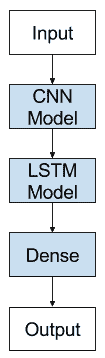### CNN Model

The Conv2D will interpret snapshots of the image (e.g. small squares) and the pooling layers will consolidate or abstract the interpretation.
We will define a Conv2D as an input layer with 2 filters and a 2 × 2 kernel to pass across the input images. The use of 2 filters was found with some experimentation and it is convention to use small kernel sizes. The Conv2D will output 2 49 × 49 pixel impressions of the input.

Convolutional layers are often immediately followed by a pooling layer. Here we use a MaxPooling2D pooling layer with a pool size of 2 × 2, which will in effect halve the size of each filter output from the previous layer, in turn outputting two 24 × 24 maps.

The pooling layer is followed by a Flatten layer to transform the [24,24,2] 3D output from the MaxPooling2D layer into a one-dimensional 1,152 element vector…

We want to apply the CNN model to each input image and pass on the output of each input image to the LSTM as a single time step.
We can achieve this by wrapping the entire CNN input model (one layer or more) in a TimeDistributed layer.

Next, we can define the LSTM elements of the model. We will use a single LSTM layer with 50 memory cells, configured after a little trial and error. The use of a TimeDistributed wrapper around the whole CNN model means that the LSTM will see 50 time steps, with each time step presenting a 1,152 element vector as input.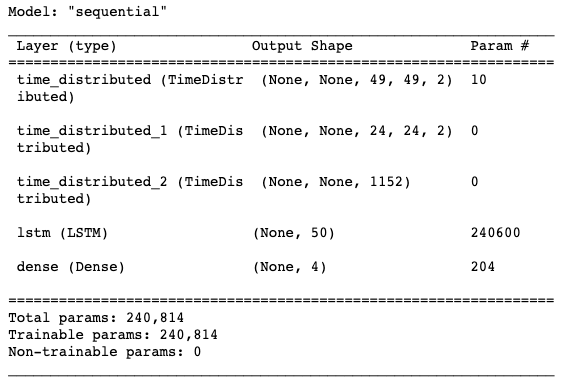### fit and evaluate the model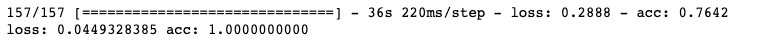### prediction